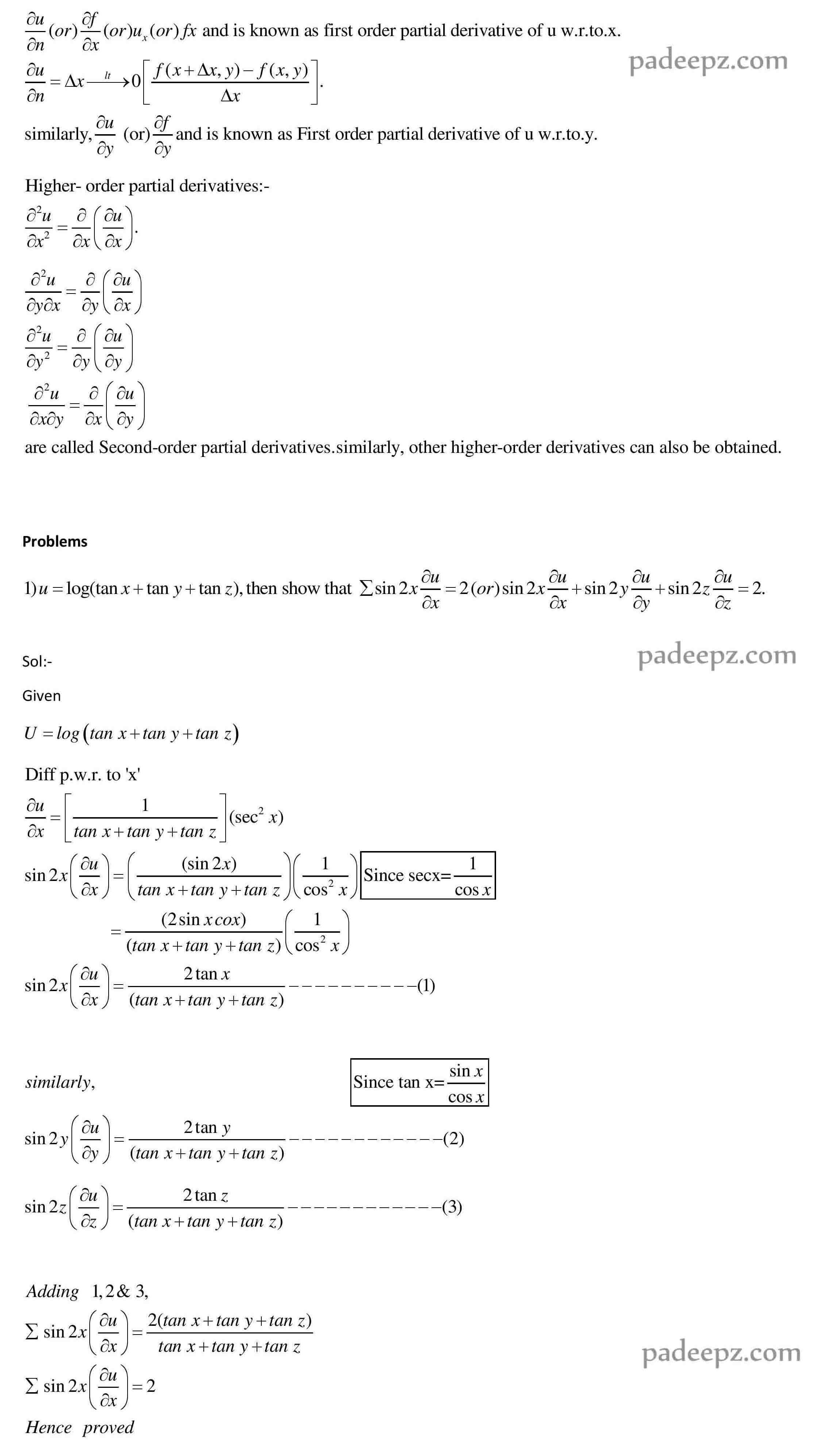# MA8151 Notes Engineering Mathematics 1

## OUTCOMES : MA8151 Notes Engineering Mathematics 1

After completing this course, students should demonstrate competency in the following skills:

 Use both the limit definition and rules of differentiation to differentiate functions.

 Apply differentiation to solve maxima and minima problems.

 Evaluate integrals both by using Riemann sums and by using the Fundamental Theorem of Calculus.

 Apply integration to compute multiple integrals, area, volume, integrals in polar
coordinates, in addition to change of order and change of variables.

 Evaluate integrals using techniques of integration, such as substitution, partial fractions and integration by parts.

 Determine convergence/divergence of improper integrals and evaluate convergent improper integrals.

 Apply various techniques in solving differential equations.

### TEXT BOOKS : MA8151 Notes Engineering Mathematics 1

1. Grewal B.S., “Higher Engineering Mathematics”, Khanna Publishers, New Delhi, 43rd Edition, 2014.

2. James Stewart, “Calculus: Early Transcendentals”, Cengage Learning, 7th Edition, New Delhi, 2015. [For Units I & III – Sections 1.1, 2.2, 2.3, 2.5, 2.7(Tangents problems only), 2.8,

3.1 to 3.6, 3.11, 4.1, 4.3, 5.1(Area problems only), 5.2, 5.3, 5.4 (excluding net change theorem), 5.5, 7.1 – 7.4 and 7.8].

#### REFERENCES : MA8151 Notes Engineering Mathematics 1

1. Anton, H, Bivens, I and Davis, S, “Calculus”, Wiley, 10th Edition, 2016.

2. Jain R.K. and Iyengar S.R.K., “Advanced Engineering Mathematics”, Narosa Publications, New Delhi, 3rd Edition, 2007.

3. Narayanan, S. and Manicavachagom Pillai, T. K., “Calculus” Volume I and II, S. Viswanathan Publishers Pvt. Ltd., Chennai, 2007.

4. Srimantha Pal and Bhunia, S.C, “Engineering Mathematics” Oxford University Press, 2015.

5. Weir, M.D and Joel Hass, “Thomas Calculus”, 12th Edition, Pearson India, 2016.

### Padeepz E-Learning Materials MA8151 Engineering Mathematics 1

Padeepz E-Learning Materials MA8151 Engineering Mathematics 1 we have provided the sample materials in this page. If you like the sample and want to buy the full subject the procedure is also provided in this page.

#### Partial derivatives

Partial Derivative Introduction:

A partial derivative of a function of several variables is the ordinary derivative with respect to one of the variables, when all the remaining variables are kept constant. Consider a function u=f(x,y). Here , u is the dependent variable and x & y are independent variables. The partial derivative of u=f(x,y) with respect to x is the ordinary derivative of u w.r.to x, keeping y constant. It is denoted bySubject name Engineering Mathematics 1 Subject Code MA8151 Semester 1 Regulation 2017 Regulation

MA8151 Syllabus Engineering Mathematics 1

MA8151 Important Questions Engineering Mathematics 1

MA8151 Question Bank Engineering Mathematics 1

## 2 Replies to “MA8151 Notes Engineering Mathematics 1 Regulation 2017 Anna University”

1.Veerasundaram says:

2.venkatesh says: